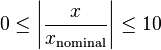# Scaling

This text is an extract of the PDF documentation.

Most nonlinear (and many linear) models cannot be solved without proper scaling of the variables. scale_self should reset the .nominal value on every real variable in need of scaling. It should then call the scale_self method on all the locally-defined (IS_A) parts of the MODEL. A proper nominal is one such that you expect at the solution$0 \le \left| \frac {x} {x_\mathrm{nominal}} \right| \le 10$

As one is dividing by the nominal value for the variable to establish its scaled value, zero is about the worst value you could choose for a nominal value.

This method should not change the .nominal values for models and variables that are received through the parameter list of the model.

Variables (like fractions), when bounded such that they cannot be too far away from 1.0 in magni- tude, probably don’t need scaling most of the time, so long as they they are also bounded away from 0.0.

Some solvers, but not all, will attempt to scale the equations and variables by heuristic matrix-based methods. This works, but inconsistently; manual user-defined scaling is generally superior. ASCEND makes scaling equations easy to do. You scale the variables, which can only be done well by knowing something about where the solution is going to be found (by being an engineer, for example).

Then ASCEND can calculate an appropriate equation-scaling by efficient symbolic methods, if relation nominal calculations are enabled in the solver. The nominal value for scaling a relation is the value of its largest additive term. There is some difference of opinion whether the largest additive term should be calculated using initial (unconverged) values of the variables in the equation or using the .nominals of the variables in the equation.

Historically, and still today, dimensionless engineering quantities are frequently used in dimensionless equations. The primary benefits of this are

• the solution can be tabulated for a huge range of physical systems that have the same single dimensionless description.
• the solution via computation is largely self-scaling and the user can assume nominals of 1.0.

Beware, however, that dimensionless quantities are also used in many dimensional equations and thus the user is ultimately responsible for handling the issue.

For more information, see the 'Equation-based Modeling' document by Piela and Westerberg.